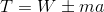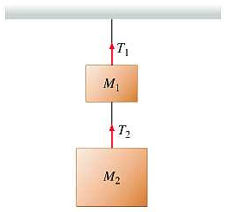# Tension Formula

## Tension Formulas - How to Calculate Tension Force

Tension refers to the pulling force transmitted axially by the means of a string, cable, chain, or similar one-dimensional continuous object, or by each end of a rod, or similar three-dimensional object; tension might also be described as the action-reaction pair of forces acting at each end of the said elements.

At the atomic level, when atoms or molecules are pulled apart from each other and gain potential energy with a restoring force still existing, the restoring force might create tension. Each end of a string or rod under such tension could pull on the object it is attached to, in order to restore the string/rod to its relaxed length.

Tension can be easily explained in the case of bodies hung from chain, cable, string etc. It is represented by (occasionally also, denoted as Ft).

If such a hung body moves vertically with an acceleration a, then;Where, W is the weight of the body and m is the mass of the body
Case (i) If the body is moving upwards, with acceleration a, the tension; T = W + ma
Case (ii) If the body is moving downwards, with acceleration a, the tension; T = W – ma
Case (iii) If the body is just suspended (not moving), the tension; T = W.
Case (iv) If the body moves up or down with uniform speed, tension; T = W

The weight of the object is W = mg.
Therefore, tension formula can be modified as:
T=m(g±a)
Where, m = mass of the body, g = acceleration due to gravity, a = acceleration of the moving body.
As tension is a force, its SI unit is newton (N).

Example:
A light and inextensible string support a body of mass of 15 kg hanging from its lower end. If the upper end of the string is firmly attached to a hook on the roof, then what is the tension in the string?
Solution:
As the body is not moving and just suspended, the tension in the string will be equal go the weight of the body. m = 15 kg
T = W = mg = 15 × 9.8 = 147 N

Example:
A monkey of mass 10 kg climbs up a light vertical string suspended from a hook with an acceleration of 2 m/s2. Find the tension in the string (take g = 10 m/s2)
Solution:
m = 10 kg, g = 10 m/s2, a = 2 m/s2
As the monkey moves up with an acceleration, the tension in the string will be equal to the apparent weight of the monkey.
i.e., T = m (g + a) = 10 (10 + 2) = 120 N

Question:
If M1 = 4 kg and M2 = 6 kg in the following figure, then T2 equals:Options:
(a) 98 N
(b) 39.2 N
(c) 58.8 N
(d) 19.6 N